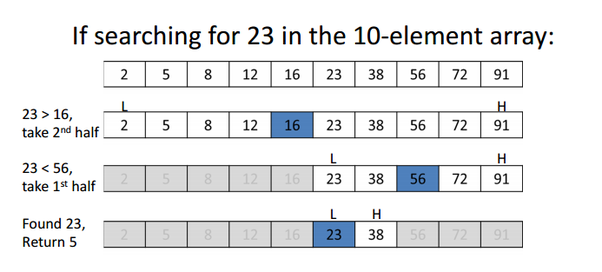## Binary Search Algorithm | Understanding & Implementing | Searching Algorithms

Intro :

The basic job of a searching algorithm is to find whether there exists the element searched by the user within some data structure .

So, there are a lot of searching algorithms out there and all of them come under either of the types :-

Sequential Search : In this type of algorithm the whole data structure is traversed in a sequence to search for the element . These are very simple and one of it's example is Linear Search which I have explained earlier .

Interval Search   : In this type of algorithm the data  must be sorted and the algorithm mainly targets the center thus dividing the search space to half segments thereby reducing the time complexity significantly and thus these are more efficient than Linear Search. An example of this is Binary Search and in this blog we are going to understand it .

Binary Search :

An efficient searching algorithm based on Divide and Conquer paradigm.

Time Complexity : O(log n)

Understanding the algorithm :

Now let's understand how the algorithms works. So, lets imagine we have an array[ ] and we want to search an element x

✔️ Always remember the first step before searching is sorting the array.

✔️ Now that the array is sorted we will target the middle element by checking whether the middle element is equal to x or not .If it is then our work is done but if it isn't then the interesting thing happens . So, now the algorithm checks whether x is less or larger than the current middle element .

✔️ Now if it is less than the middle element, so logically that means that the element x is definitely not in the right half of the middle element  as our array is sorted so, we reduce the search space to the left half and again find this segment's middle element and thus keep narrowing down and getting closer to our element x.

✔️ And exactly like that when out element x is larger than the middle element , it's just that here we discard the left half and keep repeating the process.

✔️ Now you must be wondering what will happen when the element is not present in the array . I will explain that while explaining it's implementation as it would be easy to both understand and explain there.An example of Binary search (L=left /Low, h= Right/High )

Implementation :

✔️ So, at first we have an array and and element x that we are searching for .

✔️ Now we sort the array in ascending order .

✔️ Now we declare the variables:  left = left index boundary of the current search space , right = right index boundary of the current search space and mid = index of current middle element . So at at first as the whole array is the search space : left=0 , right=array's length-1 and mid = left+(right - left)/2 .

✔️ Now we get into a while loop with condition that : left <= right which is basically always the case until we have gone through the whole segment and haven't found x in the array where we get to a condition where left becomes greater than right which will just terminate the loop and return false that we haven't found x in the array.

✔️ Well inside the while loop we find the middle index every time and then we have conditions for checking equal , less or larger between x and the middle element. :

If equal return true .
If less update right boundary : right = mid- 1  , discarding the right half .
If larger update left boundary : left = mid + 1  , discarding the left half .

The Code :

From the Classical Algorithm Reposititory. Do give a star ! 🌟

So, that's how in half of the time as compared to linear search we perform the search operation efficiently using Binary Search  .